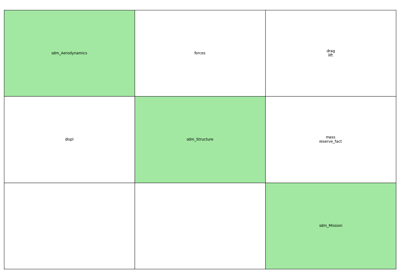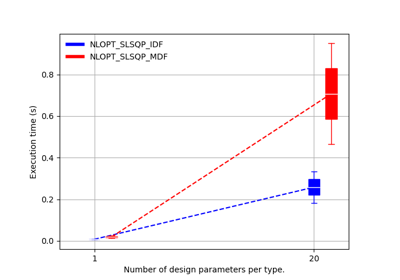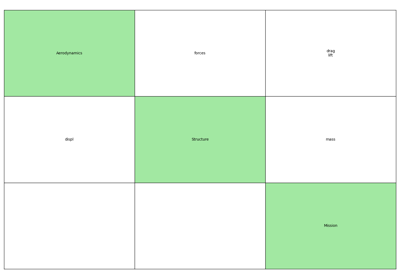gemseo / problems / aerostructure

# aerostructure_design_space module¶

## Aerostructure MDO problem’s Design Space¶

class gemseo.problems.aerostructure.aerostructure_design_space.AerostructureDesignSpace[source]

Bases: DesignSpace

AerostructureDesignSpace creates the DesignSpace of the Aerostructure problem whose MDODiscipline are Aerodynamics, Structure and Mission.

• thick_airfoils belongs to [5., 25.], with initial value equal to 15.

• thick_panels belongs to [1., 20.], with initial value equal to 3.

• sweep belongs to [10., 35.], with initial value equal to 25.

• drag belongs to [100., 1000.], with initial value equal to 340.

• forces belongs to [-1000., 1000.], with initial value equal to 400.

• lift belongs to [0.1, 1.0], with initial value equal to 0.5

• mass belongs to [100000., 500000.], with initial value equal to 100000.

• reserve_fact belongs to [1000., 1000.], with initial value equal to 0.

Constructor.

add_variable(name, size=1, var_type=DesignVariableType.FLOAT, l_b=None, u_b=None, value=None)

Add a variable to the design space.

Parameters:
• name (str) – The name of the variable.

• size (int) –

The size of the variable.

By default it is set to 1.

• var_type (VarType) –

Either the type of the variable or the types of its components.

By default it is set to FLOAT.

• l_b (float | ndarray | None) – The lower bound of the variable. If None, use $$-\infty$$.

• u_b (float | ndarray | None) – The upper bound of the variable. If None, use $$+\infty$$.

• value (float | ndarray | None) – The default value of the variable. If None, do not use a default value.

Raises:

ValueError – Either if the variable already exists or if the size is not a positive integer.

Return type:

None

array_to_dict(x_array)

Convert a design array into a dictionary indexed by the variables names.

Parameters:

x_array (ndarray) – A design value expressed as a NumPy array.

Returns:

The design value expressed as a dictionary of NumPy arrays.

Return type:
check()

Check the state of the design space.

Raises:

ValueError – If the design space is empty.

Return type:

None

check_membership(x_vect, variable_names=None)

Check whether the variables satisfy the design space requirements.

Parameters:
• x_vect (Mapping[str, ndarray] | ndarray) – The values of the variables.

• variable_names (Sequence[str] | None) – The names of the variables. If None, use the names of the variables of the design space.

Raises:

ValueError – Either if the dimension of the values vector is wrong, if the values are not specified as an array or a dictionary, if the values are outside the bounds of the variables or if the component of an integer variable is not an integer.

Return type:

None

clear() None.  Remove all items from D.
dict_to_array(design_values, variable_names=None)

Convert a point as dictionary into an array.

Parameters:
• design_values (dict[str, numpy.ndarray]) – The design point to be converted.

• variable_names (Optional[Iterable[str]]) – The variables to be considered. If None, use the variables of the design space.

Returns:

The point as an array.

Return type:

ndarray

export_hdf(file_path, append=False)

Export the design space to an HDF file.

Parameters:
• file_path (str | Path) – The path to the file to export the design space.

• append (bool) –

If True, appends the data in the file.

By default it is set to False.

Return type:

None

Export the design space to a text file.

Parameters:
Return type:

None

extend(other)

Extend the design space with another design space.

Parameters:

other (DesignSpace) – The design space to be appended to the current one.

Return type:

None

filter(keep_variables, copy=False)

Filter the design space to keep a subset of variables.

Parameters:
• keep_variables (str | Iterable[str]) – The names of the variables to be kept.

• copy (bool) –

If True, then a copy of the design space is filtered, otherwise the design space itself is filtered.

By default it is set to False.

Returns:

Either the filtered original design space or a copy.

Raises:

ValueError – If the variable is not in the design space.

Return type:

DesignSpace

filter_dim(variable, keep_dimensions)

Filter the design space to keep a subset of dimensions for a variable.

Parameters:
• variable (str) – The name of the variable.

• keep_dimensions (Iterable[int]) – The dimensions of the variable to be kept, between $$0$$ and $$d-1$$ where $$d$$ is the number of dimensions of the variable.

Returns:

The filtered design space.

Raises:

ValueError – If a dimension is unknown.

Return type:

DesignSpace

get(k[, d]) D[k] if k in D, else d.  d defaults to None.
get_active_bounds(x_vec=None, tol=1e-08)

Determine which bound constraints of a design value are active.

Parameters:
• x_vec (ndarray | None) – The design value at which to check the bounds. If None, use the current design value.

• tol (float) –

The tolerance of comparison of a scalar with a bound.

By default it is set to 1e-08.

Returns:

Whether the components of the lower and upper bound constraints are active, the first returned value representing the lower bounds and the second one the upper bounds, e.g.

({'x': array(are_x_lower_bounds_active),
'y': array(are_y_lower_bounds_active)},
{'x': array(are_x_upper_bounds_active),
'y': array(are_y_upper_bounds_active)}
)


where:

are_x_lower_bounds_active = [True, False]
are_x_upper_bounds_active = [False, False]
are_y_lower_bounds_active = [False]
are_y_upper_bounds_active = [True]


Return type:

tuple[dict[str, ndarray], dict[str, ndarray]]

get_current_value(variable_names=None, complex_to_real=False, as_dict=False, normalize=False)

Return the current design value.

If the names of the variables are empty then an empty data is returned.

Parameters:
• variable_names (Sequence[str] | None) – The names of the design variables. If None, use all the design variables.

• complex_to_real (bool) –

Whether to cast complex numbers to real ones.

By default it is set to False.

• as_dict (bool) –

Whether to return the current design value as a dictionary of the form {variable_name: variable_value}.

By default it is set to False.

• normalize (bool) –

Whether to normalize the design values in $$[0,1]$$ with the bounds of the variables.

By default it is set to False.

Returns:

The current design value.

Raises:

ValueError – If names in variable_names are not in the design space.

Return type:

ndarray | dict[str, ndarray]

Warning

For performance purposes, get_current_value() does not return a copy of the current value. This means that modifying the returned object will make the DesignSpace inconsistent (the current design value stored as a NumPy array and the current design value stored as a dictionary of NumPy arrays will be different). To modify the returned object without impacting the DesignSpace, you shall copy this object and modify the copy.

To modify the current value, please use set_current_value() or set_current_variable().

get_indexed_var_name(variable_name)

Create the names of the components of a variable.

If the size of the variable is equal to 1, this method returns the name of the variable. Otherwise, it concatenates the name of the variable, the separator DesignSpace.SEP and the index of the component.

Parameters:

variable_name (str) – The name of the variable.

Returns:

The names of the components of the variable.

Return type:

str | list[str]

get_indexed_variables_names()

Create the names of the components of all the variables.

If the size of the variable is equal to 1, this method uses its name. Otherwise, it concatenates the name of the variable, the separator DesignSpace.SEP and the index of the component.

Returns:

The name of the components of all the variables.

Return type:

list[str]

get_lower_bound(name)

Return the lower bound of a variable.

Parameters:

name (str) – The name of the variable.

Returns:

The lower bound of the variable (possibly infinite).

Return type:

ndarray

get_lower_bounds(variable_names=None)

Generate an array of the variables’ lower bounds.

Parameters:

variable_names (Sequence[str] | None) – The names of the variables of which the lower bounds are required. If None, use the variables of the design space.

Returns:

The lower bounds of the variables.

Return type:

ndarray

get_pretty_table(fields=None, with_index=False)

Build a tabular view of the design space.

Parameters:
• fields (Sequence[str] | None) – The name of the fields to be exported. If None, export all the fields.

• with_index (bool) –

Whether to show index of names for arrays. This is ignored for scalars.

By default it is set to False.

Returns:

A tabular view of the design space.

Return type:

PrettyTable

get_size(name)

Get the size of a variable.

Parameters:

name (str) – The name of the variable.

Returns:

The size of the variable, None if it is not known.

Return type:

int | None

get_type(name)

Return the type of a variable.

Parameters:

name (str) – The name of the variable.

Returns:

The type of the variable, None if it is not known.

Return type:

str | None

get_upper_bound(name)

Return the upper bound of a variable.

Parameters:

name (str) – The name of the variable.

Returns:

The upper bound of the variable (possibly infinite).

Return type:

ndarray

get_upper_bounds(variable_names=None)

Generate an array of the variables’ upper bounds.

Parameters:

variable_names (Sequence[str] | None) – The names of the variables of which the upper bounds are required. If None, use the variables of the design space.

Returns:

The upper bounds of the variables.

Return type:

ndarray

get_variables_indexes(variable_names)

Return the indexes of a design array corresponding to the variables names.

Parameters:

variable_names (Iterable[str]) – The names of the variables.

Returns:

The indexes of a design array corresponding to the variables names.

Return type:

ndarray

has_current_value()

Check if each variable has a current value.

Returns:

Whether the current design value is defined for all variables.

Return type:

bool

has_integer_variables()

Check if the design space has at least one integer variable.

Returns:

Whether the design space has at least one integer variable.

Return type:

bool

import_hdf(file_path)

Import a design space from an HDF file.

Parameters:

file_path (str | Path) – The path to the file containing the description of a design space.

Return type:

None

initialize_missing_current_values()

Initialize the current values of the design variables when missing.

Use:

• the center of the design space when the lower and upper bounds are finite,

• the lower bounds when the upper bounds are infinite,

• the upper bounds when the lower bounds are infinite,

• zero when the lower and upper bounds are infinite.

Return type:

None

items() a set-like object providing a view on D's items
keys() a set-like object providing a view on D's keys

This method is based on the chain rule:

$\frac{df(x)}{dx} = \frac{df(x)}{dx_u}\frac{dx_u}{dx} = \frac{df(x)}{dx_u}\frac{1}{u_b-l_b}$

where $$x_u = \frac{x-l_b}{u_b-l_b}$$ is the normalized input vector, $$x$$ is the unnormalized input vector and $$l_b$$ and $$u_b$$ are the lower and upper bounds of $$x$$.

$\frac{df(x)}{dx_u} = (u_b-l_b)\frac{df(x)}{dx}$

where $$\frac{df(x)}{dx}$$ is the unnormalized one.

Parameters:

g_vect (ndarray) – The gradient to be normalized.

Returns:

Return type:

ndarray

normalize_vect(x_vect, minus_lb=True, out=None)

Normalize a vector of the design space.

If minus_lb is True:

$x_u = \frac{x-l_b}{u_b-l_b}$

where $$l_b$$ and $$u_b$$ are the lower and upper bounds of $$x$$.

Otherwise:

$x_u = \frac{x}{u_b-l_b}$

Unbounded variables are not normalized.

Parameters:
• x_vect (ndarray) – The values of the design variables.

• minus_lb (bool) –

If True, remove the lower bounds at normalization.

By default it is set to True.

• out (ndarray | None) – The array to store the normalized vector. If None, create a new array.

Returns:

The normalized vector.

Return type:

ndarray

pop(k[, d]) v, remove specified key and return the corresponding value.

If key is not found, d is returned if given, otherwise KeyError is raised.

popitem() (k, v), remove and return some (key, value) pair

as a 2-tuple; but raise KeyError if D is empty.

project_into_bounds(x_c, normalized=False)

Project a vector onto the bounds, using a simple coordinate wise approach.

Parameters:
• normalized (bool) –

If True, then the vector is assumed to be normalized.

By default it is set to False.

• x_c (ndarray) – The vector to be projected onto the bounds.

Returns:

The projected vector.

Return type:

ndarray

Create a design space from a text file.

Parameters:
• input_file (str | Path) – The path to the file.

• header (Iterable[str] | None) – The names of the fields saved in the file. If None, read them in the file.

Returns:

The design space read from the file.

Raises:

ValueError – If the file does not contain the minimal variables in its header.

Return type:

DesignSpace

remove_variable(name)

Remove a variable from the design space.

Parameters:

name (str) – The name of the variable to be removed.

Return type:

None

rename_variable(current_name, new_name)

Rename a variable.

Parameters:
• current_name (str) – The name of the variable to rename.

• new_name (str) – The new name of the variable.

Return type:

None

round_vect(x_vect, copy=True)

Round the vector where variables are of integer type.

Parameters:
• x_vect (ndarray) – The values to be rounded.

• copy (bool) –

Whether to round a copy of x_vect.

By default it is set to True.

Returns:

The rounded values.

Return type:

ndarray

set_current_value(value)

Set the current design value.

Parameters:

value (ndarray | Mapping[str, ndarray] | OptimizationResult) – The value of the current design.

Raises:
Return type:

None

set_current_variable(name, current_value)

Set the current value of a single variable.

Parameters:
• name (str) – The name of the variable.

• current_value (ndarray) – The current value of the variable.

Return type:

None

set_lower_bound(name, lower_bound)

Set the lower bound of a variable.

Parameters:
• name (str) – The name of the variable.

• lower_bound (ndarray | None) – The value of the lower bound.

Raises:

ValueError – If the variable does not exist.

Return type:

None

set_upper_bound(name, upper_bound)

Set the upper bound of a variable.

Parameters:
• name (str) – The name of the variable.

• upper_bound (ndarray | None) – The value of the upper bound.

Raises:

ValueError – If the variable does not exist.

Return type:

None

setdefault(k[, d]) D.get(k,d), also set D[k]=d if k not in D
to_complex()

Cast the current value to complex.

Return type:

None

transform_vect(vector, out=None)

Map a point of the design space to a vector with components in $$[0,1]$$.

Parameters:
• vector (ndarray) – A point of the design space.

• out (ndarray | None) – The array to store the transformed vector. If None, create a new array.

Returns:

A vector with components in $$[0,1]$$.

Return type:

ndarray

This method is based on the chain rule:

$\frac{df(x)}{dx} = \frac{df(x)}{dx_u}\frac{dx_u}{dx} = \frac{df(x)}{dx_u}\frac{1}{u_b-l_b}$

where $$x_u = \frac{x-l_b}{u_b-l_b}$$ is the normalized input vector, $$x$$ is the unnormalized input vector, $$\frac{df(x)}{dx_u}$$ is the unnormalized gradient $$\frac{df(x)}{dx}$$ is the normalized one, and $$l_b$$ and $$u_b$$ are the lower and upper bounds of $$x$$.

Parameters:

g_vect (ndarray) – The gradient to be unnormalized.

Returns:

Return type:

ndarray

unnormalize_vect(x_vect, minus_lb=True, no_check=False, out=None)

Unnormalize a normalized vector of the design space.

If minus_lb is True:

$x = x_u(u_b-l_b) + l_b$

where $$x_u$$ is the normalized input vector, $$x$$ is the unnormalized input vector and $$l_b$$ and $$u_b$$ are the lower and upper bounds of $$x$$.

Otherwise:

$x = x_u(u_b-l_b)$
Parameters:
• x_vect (ndarray) – The values of the design variables.

• minus_lb (bool) –

Whether to remove the lower bounds at normalization.

By default it is set to True.

• no_check (bool) –

Whether to check if the components are in $$[0,1]$$.

By default it is set to False.

• out (ndarray | None) – The array to store the unnormalized vector. If None, create a new array.

Returns:

The unnormalized vector.

Return type:

ndarray

untransform_vect(vector, no_check=False, out=None)

Map a vector with components in $$[0,1]$$ to the design space.

Parameters:
• vector (ndarray) – A vector with components in $$[0,1]$$.

• no_check (bool) –

Whether to check if the components are in $$[0,1]$$.

By default it is set to False.

• out (ndarray | None) – The array to store the untransformed vector. If None, create a new array.

Returns:

A point of the variables space.

Return type:

ndarray

update([E, ]**F) None.  Update D from mapping/iterable E and F.

If E present and has a .keys() method, does: for k in E: D[k] = E[k] If E present and lacks .keys() method, does: for (k, v) in E: D[k] = v In either case, this is followed by: for k, v in F.items(): D[k] = v

values() an object providing a view on D's values
AVAILABLE_TYPES = [<DesignVariableType.FLOAT: 'float'>, <DesignVariableType.INTEGER: 'integer'>]
DESIGN_SPACE_GROUP = 'design_space'
FLOAT = 'float'
INTEGER = 'integer'
LB_GROUP = 'l_b'
MINIMAL_FIELDS = ['name', 'lower_bound', 'upper_bound']
NAMES_GROUP = 'names'
NAME_GROUP = 'name'
SEP = '!'
SIZE_GROUP = 'size'
TABLE_NAMES = ['name', 'lower_bound', 'value', 'upper_bound', 'type']
UB_GROUP = 'u_b'
VALUE_GROUP = 'value'
VAR_TYPE_GROUP = 'var_type'
dimension: int

The total dimension of the space, corresponding to the sum of the sizes of the variables.

name: str | None

The name of the space.

normalize: dict[str, ndarray]

The normalization policies of the variables components indexed by the variables names; if True, the component can be normalized.

variables_names: list[str]

The names of the variables.

variables_sizes: dict[str, int]

The sizes of the variables.

variables_types: dict[str, ndarray]

The types of the variables components, which can be any DesignSpace.DesignVariableType.

## Examples using AerostructureDesignSpace¶Scalable problem

Scalable problemScalable study

Scalable studyMDO formulations for a toy example in aerostructure

MDO formulations for a toy example in aerostructure